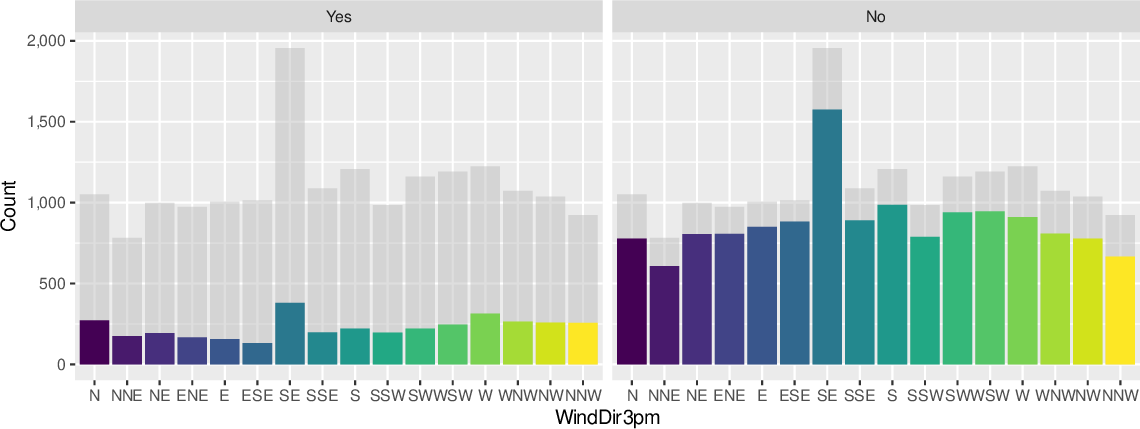Data Science Desktop Survival Guide by Graham WilliamsDesktop Survival Project Home Preface Data Science Introducing R R Constructs R Tasks R Strings R Read, Write, and Create Data Template Data Exploration Data Wrangling Data Visualisation Statistics ML Template ML Scenarios ML Activities ML Applications ML Algorithms Cluster Analysis Decision Trees Computer Vision Graph Data Privacy Literate Data Science Coding with Style Resources Bibliography Index

## Bar Chart Faceted Background

20200823ds %>%   sample_frac(0.1) %T>%   {assign("norain", . %>% select(-rain_tomorrow), 1)} %>%   mutate(rain_tomorrow=factor(rain_tomorrow,                               levels=(rain_tomorrow %>% unique() %>%                                         sort() %>% rev()))) %>%   ggplot(aes(x=wind_dir_3pm, fill=wind_dir_3pm)) +   geom_bar(data=norain, fill="gray", alpha=0.5) +   geom_bar() +   facet_wrap(~ rain_tomorrow) +   labs(x=vnames["wind_dir_3pm"], y="Count") +   scale_y_continuous(labels=comma) +   theme(legend.position="none") Using a faceted bar chart we can compare the individual charts with the global chart shown as a gray background. Take a 10% sample. Remove the target column and save. Swap the order of No/Yes in the target column. Plot the wind direction. Add a grey layer with the non-target variable dataset. Add normal bar layer. Facet. Labels will have commas. Turn the legend off.